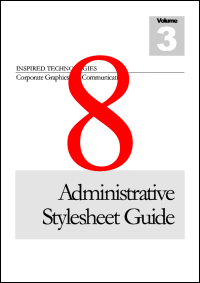Deletion ExampleThis example shows how to delete pages from a PDF document.Setup

 First we create an ABCpdf Doc object and read our source document. We store the number of pages we're going to delete - we're going to delete all but one. [C#] ```Doc theDoc = new Doc(); theDoc.Read(Server.MapPath("../mypics/sample.pdf")); int theCount = theDoc.PageCount - 1;``` [Visual Basic] ```Dim theDoc As Doc = New Doc() theDoc.Read(Server.MapPath("../mypics/sample.pdf")) Dim theCount As Integer = theDoc.PageCount - 1```Delete

 We go round a loop deleting the second page each time. [C#] ```for (int i = 0; i < theCount; i++) {   theDoc.PageNumber = 2;   theDoc.Delete(theDoc.Page); }``` [Visual Basic] ```For i As Integer = 1 to theCount   theDoc.PageNumber = 2   theDoc.Delete(theDoc.Page) Next```Save

 We add some text to the PDF so that we know how many pages we've deleted. Finally we save the PDF. [C#] ```theDoc.FontSize = 500; theDoc.Color.String = "255 0 0"; theDoc.TextStyle.HPos = 0.5; theDoc.TextStyle.VPos = 0.3; theDoc.AddText(theCount.ToString()); theDoc.Save(Server.MapPath("deletion.pdf")); theDoc.Clear();``` [Visual Basic] ```theDoc.FontSize = 500 theDoc.Color.String = "255 0 0" theDoc.TextStyle.HPos = 0.5 theDoc.TextStyle.VPos = 0.3 theDoc.AddText(theCount.ToString()) theDoc.Save(Server.MapPath("deletion.pdf")) theDoc.Clear()```Resultsdeletion.pdf